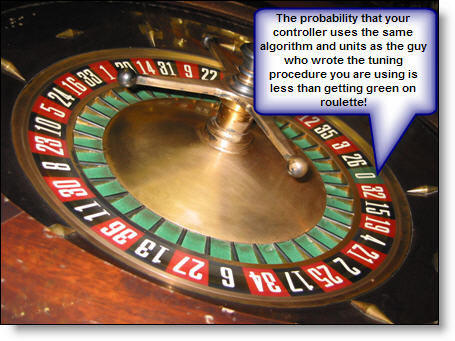# Pid Tuning Blueprint

## Is there an Industry Standard for PID Controllers?

One day, a plant engineer, replaced his ageing controllers. Even though he used all the same settings on the new controllers, the retrofitted loops went dramatically unstable when placed in Auto mode.

He tried to tune these controllers using exactly the same technique with which he had tuned the old ones. The loops seemed to get more unstable.

This all too common situation is the result of two manufacturers using completely different PID algorithms.

Believe it or not, there are no adhered to industry standards that define how a PID controller should work, what algorithm it uses and what the various configurable constants should be called.

The result is that you can upgrade a controller to a new model or manufacturer, copy the exact P, I and D settings over, and end up with a completely out-of-control process.

We live in a world where all vendors implement the PID control algorithm with different degrees of simplicity and functionality.

This means that it is essential to first identify and then understand the controller type you are tuning.

The variations that we need to identify, because they will affect the tuning, are summarized in this table:

ALGORITHM TYPE is either: "P Constant": can be either: "I constant" : unites can be either "D Constant" : unites can be either
I = Ideal G = Proportional Gain S/R = Seconds per Repeat S = Secounds
S = Series % = Proportional Band S/R = Repeats per Second M = Minutes
P = Parallel   M/R = Minutes per Repeat
R/M = Repeats per Minute

That means that there are 3 x 2 x 4 x 2 = 48 different combinations of units and algorithms that any one controller can use!

What are the chances that when PID loop tuning, the controller you are using has the same units and algorithms as the next guy? A bit less than getting green on the roulette table!This means, of course, that you have to know:

• which Algorithm your tuning rules are designed for
• and which units it gives you the answers in

And then convert the tuning constants to your algorithm and your units.

The PID Tuning Blueprint walks you step-by-step through:

• How to find out what algorithm your controller uses.
• How to convert your calculated P I and D constants so that they work with your controller.
• How to convert your constants to the units that your controller uses.

And the upgraded 'pro package' version of the toolkit lets you use PID Simulation to make any learners' mistakes at your desk, not on the plant!